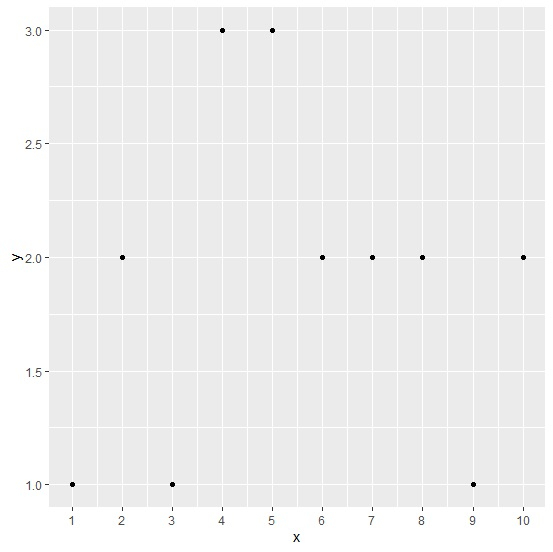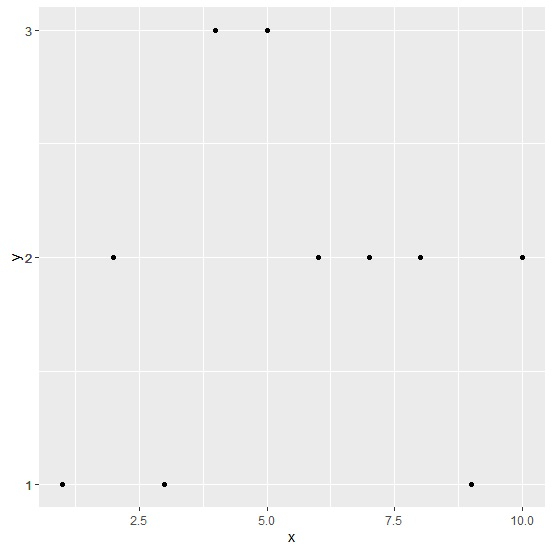# How to represent all values of X-axis or Y-axis on the graph in R using ggplot2 package?

If we have many unique elements or repeated in a column of an R data frame and create a graph using that column, either on X-axis or Y-axis then R automatically choses the axes labels, this might not display all the unique values of the column in the plot. Therefore, we can use scale_x_continuous function or scale_y_continuous function with labels depending on our requirement to display the column values.

Consider the below data frame −

## Example

Live Demo

x<-1:10
y<-rpois(10,2)
df<-data.frame(x,y)
df

## Output

x y
1 1 1
2 2 1
3 3 5
4 4 3
5 5 3
6 6 0
7 7 2
8 8 5
9 9 2
10 10 4

Loading ggplot2 package and creating a point chart between x and y by displaying all values of x axis −

## Example

library(ggplot2) ggplot(df,aes(x,y))+geom_point()+scale_x_continuous(labels=as.character(x),breaks=x)Creating a point chart between x and y by displaying all values of Y axis −

## Example

ggplot(df,aes(x,y))+geom_point()+scale_y_continuous(labels=as.character(y),breaks=y)